The PHREG Procedure
 The Multiplicative Hazards Model

Consider a set ofsubjects such that the counting process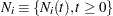for theth subject represents the number of observed events experienced over time. The sample paths of the process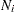are step functions with jumps of size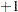, with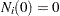. Letdenote the vector of unknown regression coefficients. The multiplicative hazards function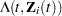foris given by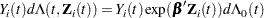where

•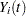indicates whether theth subject is at risk at time(specifically,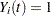if at risk and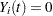otherwise)

•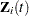is the vector of explanatory variables for theth subject at time•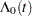is an unspecified baseline hazard function

Refer to Fleming and Harrington (1991) and Andersen et al. (1992). The Cox model is a special case of this multiplicative hazards model, whereuntil the first event or censoring, andthereafter.

The partial likelihood forindependent triplets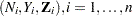, has the form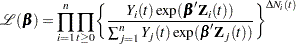where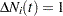if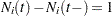, and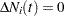otherwise.Previous Page | Next Page | Top of Page